Algebra 1 7-8 Complete Lesson: Geometric Sequences
starstarstarstarstarstarstarstarstarstar
4.5 (1 rating)
by Matthew Richardson
| 31 Questions
Note from the author:
A complete formative lesson with embedded slideshow, mini lecture screencasts, checks for understanding, practice items, mixed review, and reflection. I create these assignments to supplement each lesson of Pearson's Common Core Edition Algebra 1, Algebra 2, and Geometry courses. See also mathquest.net and twitter.com/mathquestEDU.The outlined content above was added from outside of Formative.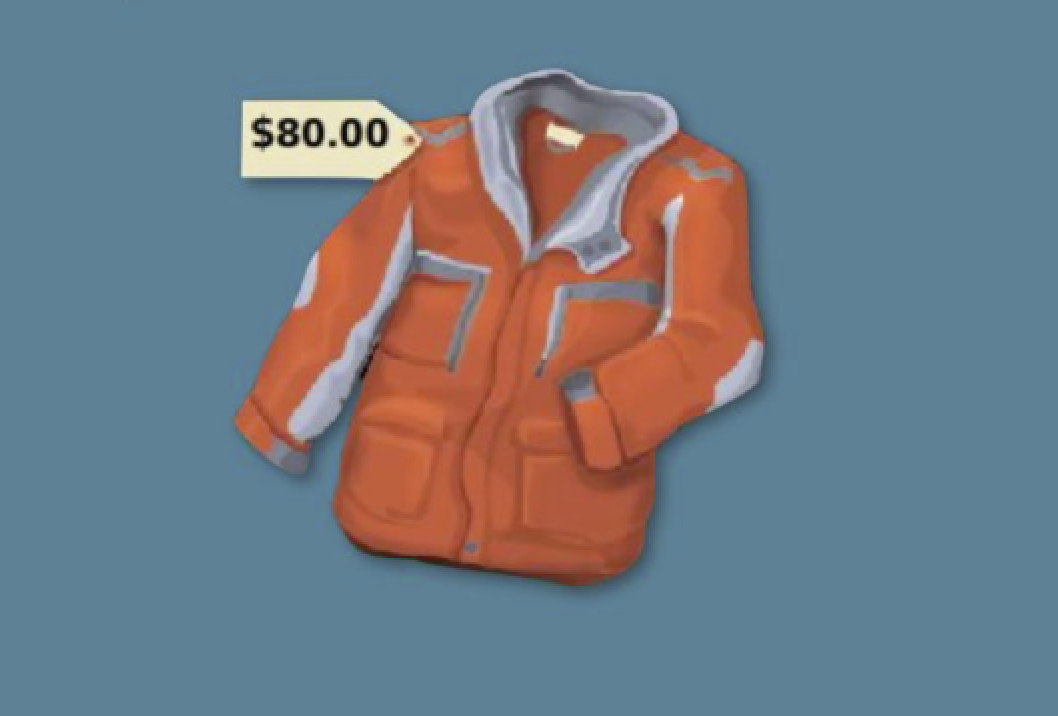1
1
10 pts
Solve It! Imagine working part time at a clothing store. Each week a coat doesn't sell, its price is marked down 20%. What will the sale price for the coat be after three weeks of not selling? Enter the dollar amount in this form: \$71.23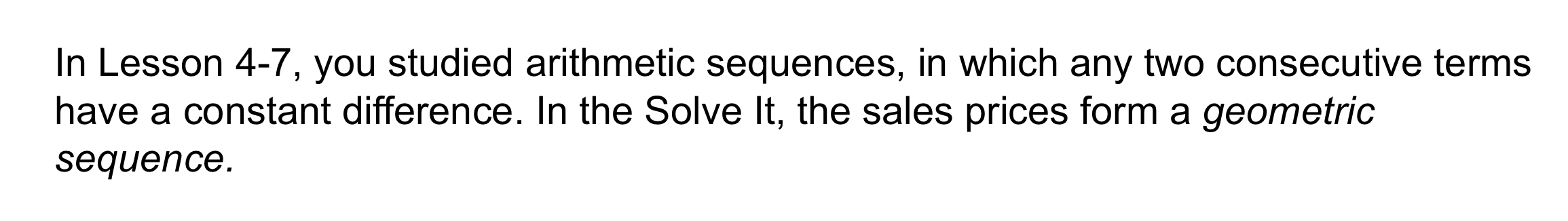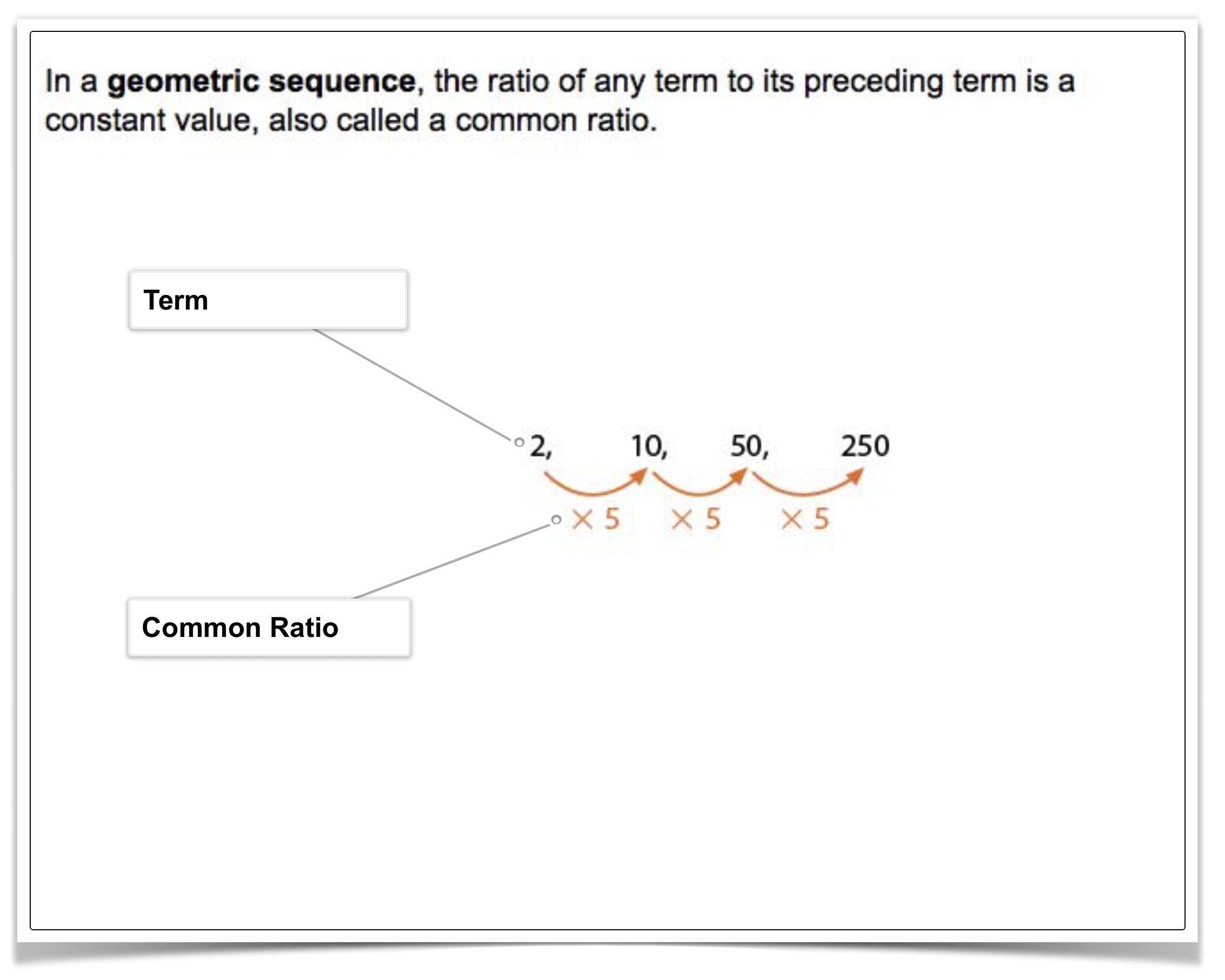2
2
10 pts
Problem 1 Got It?
A
B
C
D3
3
10 pts
Problem 1 Got It?
A
B
C
D4
4
10 pts
Problem 1 Got It?
A
B
C
D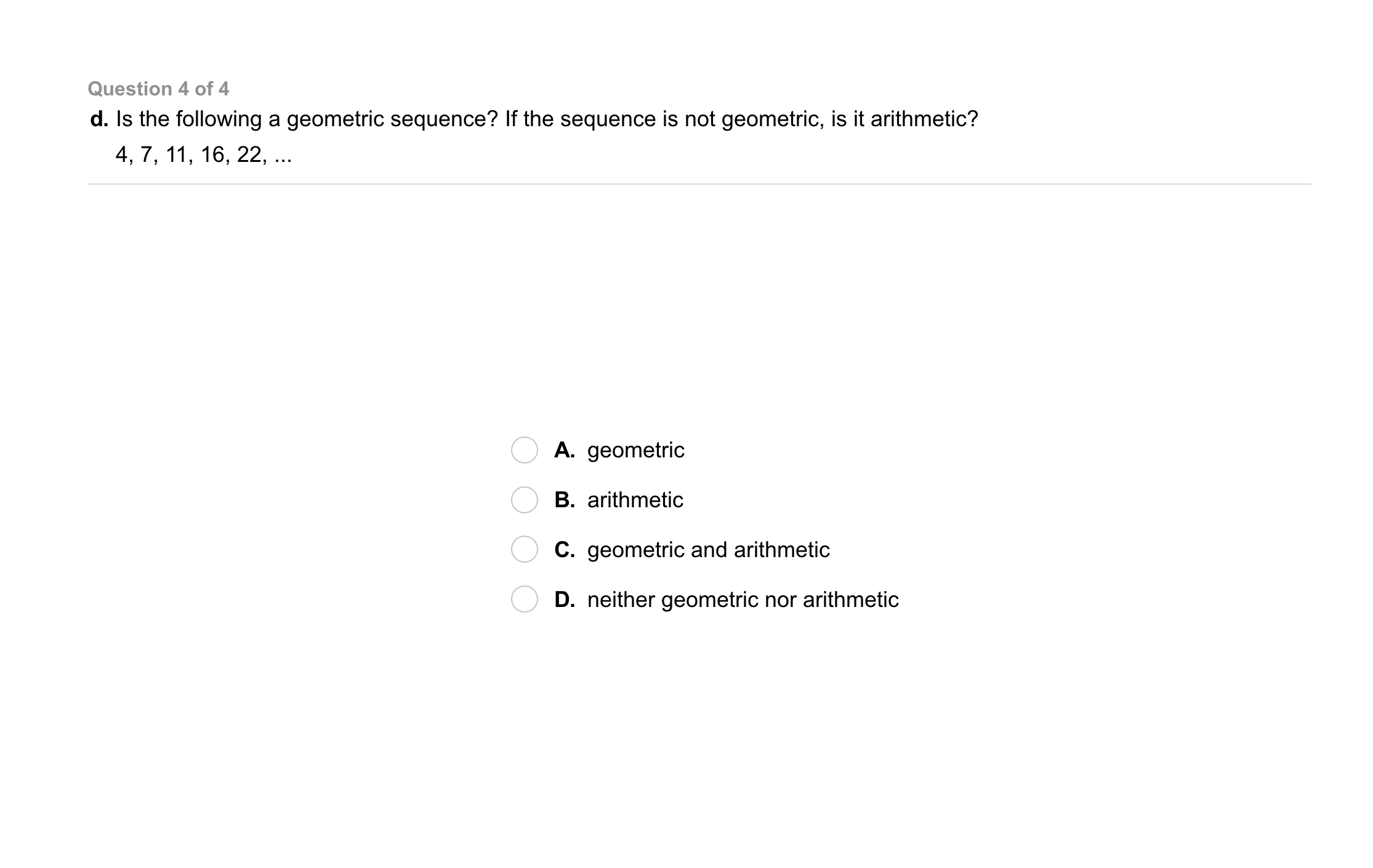5
5
10 pts
Problem 1 Got It?
A
B
C
D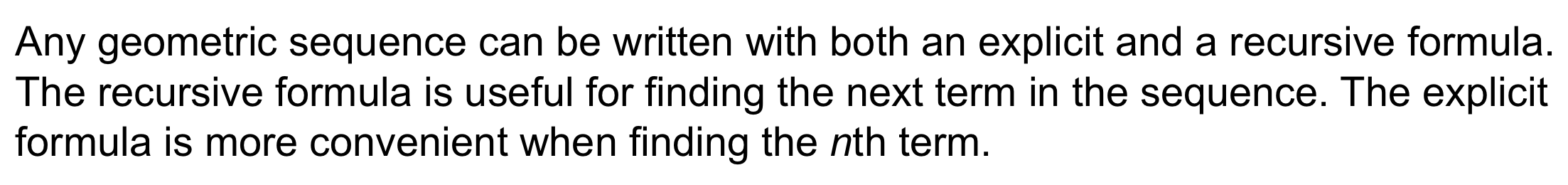6
6
10 pts
Problem 2 Got It?
A
B
C
D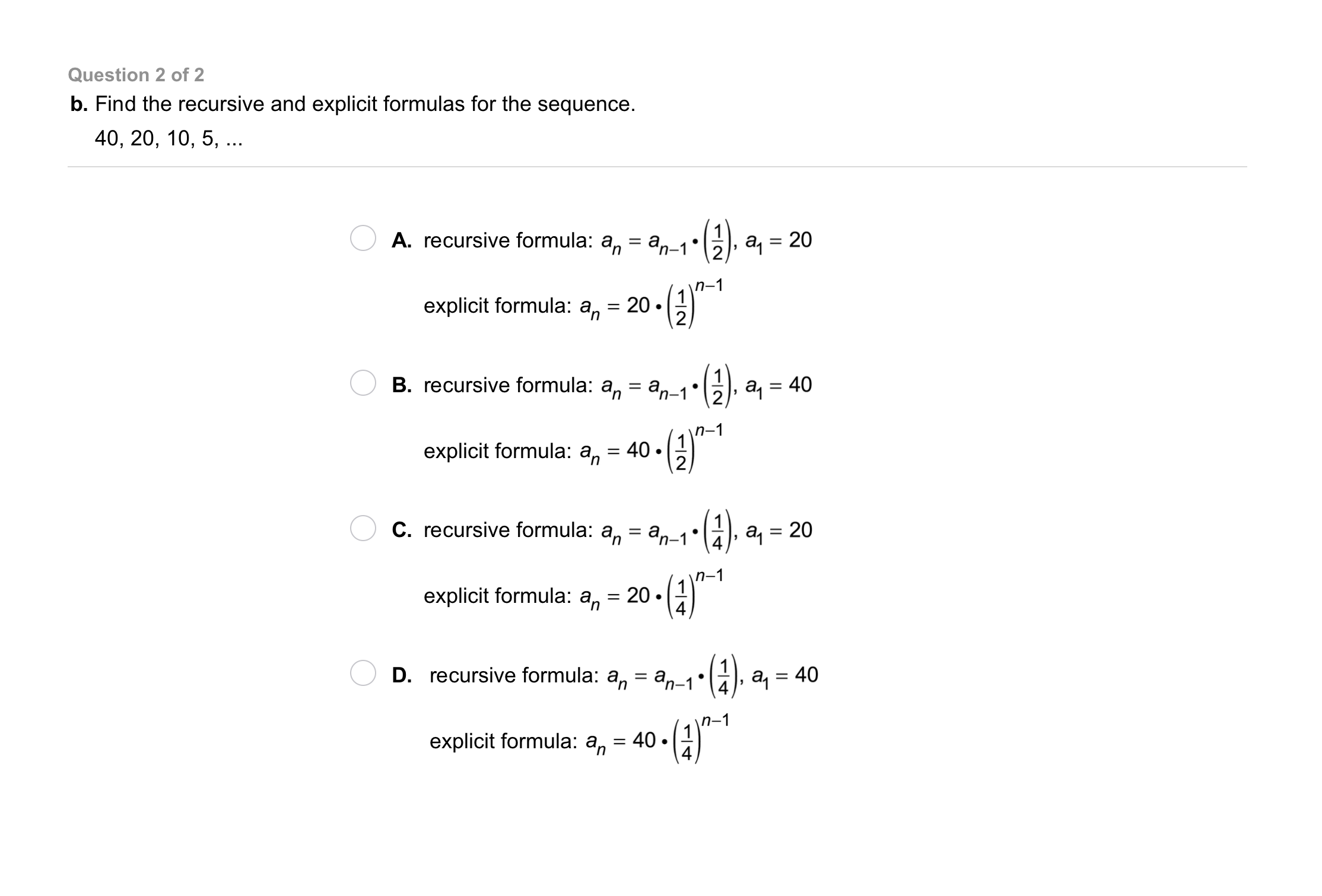7
7
10 pts
Problem 2 Got It?
A
B
C
D
8
10 pts
Problem 3 Got It? Write a recursive formula and an explicit formula for the sequence. Find the 8th term of the sequence.

9
10 pts
Problem 3 Got It? Write a recursive formula and an explicit formula for the sequence. Find the 8th term of the sequence.10
20 pts
Problem 4 Got It? A geometric sequence has an initial value of 2 and a common ratio of 3. Write a function to represent the sequence. Graph the function. Include all relevant graph detail.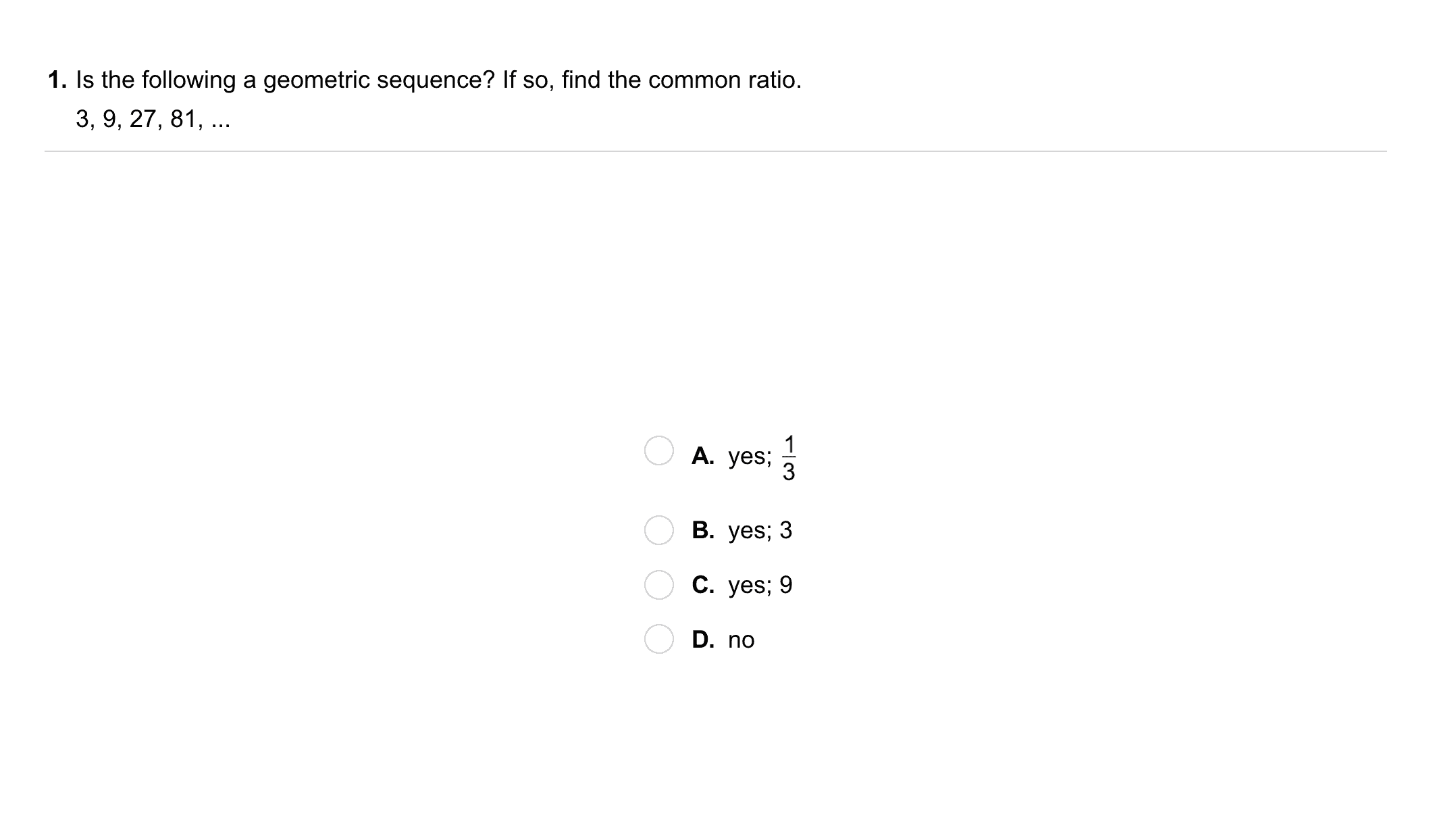11
11
10 pts
A
B
C
D
12
10 pts
Is the following a geometric sequence? If so, find the common ratio.
Write your answer in one of these formats: "yes; 1/2" or "no"13
13
10 pts
A
B
C
D
14
10 pts
Vocabulary Review: Write the ratio in simplest form.
Use fraction form.
15
40 pts
Write the explicit and recursive formulas for each sequence on the canvas.16
10 pts
Error Analysis: A friend provides the following recursive formula for the geometric sequence 1, -1, 1, -1, 1, ...
Explain your friend's error and give the correct recursive formula for the sequence.
17
10 pts
Critical Thinking: Describe the similarities and differences between arithmetic and geometric sequences.18
10 pts
Review Lesson 2-10: The value 25 is increased by 50%. What is the new value? Enter only an exact decimal for your response.
19
10 pts
Review Lesson 2-3: Match each solution with the appropriate equation(s).
• x = -2
• x = 16
• x = -8
• 3x + 7 = 2x - 1
• x ÷ 2 + 5 = -x + 2
• 4(x - 3) = 20 + 2x
• x + -8 = 2x
20
10 pts
Review Lesson 2-10: A price changes from \$40 to \$70. What is the percent change?
133%
175%
75%
43%
21
10 pts
Review Lesson 1-8: Simplify the expression.

22
10 pts
Review Lesson 1-8: Simplify the expression.

23
10 pts
Review Lesson 1-8: Simplify the expression.

24
10 pts
Review Lesson 4-7: Write the recursive and explicit formulas for each arithmetic sequence.

0, 9, 18, 27,...

5, 3, 1, -1,...

-7, -3, 1, 5,...25
10 pts
Vocabulary Review: Complete the sentence.
• greatest common factor
• least common multiple
• To write a ratio in simplest form, divide the numerator and denominator by their __?__.
26
10 pts
Vocabulary Review: Write the ratio in simplest form.
Use this format: 1/2
1/4
1/2
3/4
4/3
27
10 pts
Vocabulary Review: Write the ratio in simplest form.
Use this format: 1/2
28
10 pts
Vocabulary Review: Write the ratio in simplest form.
Use this format: 1/2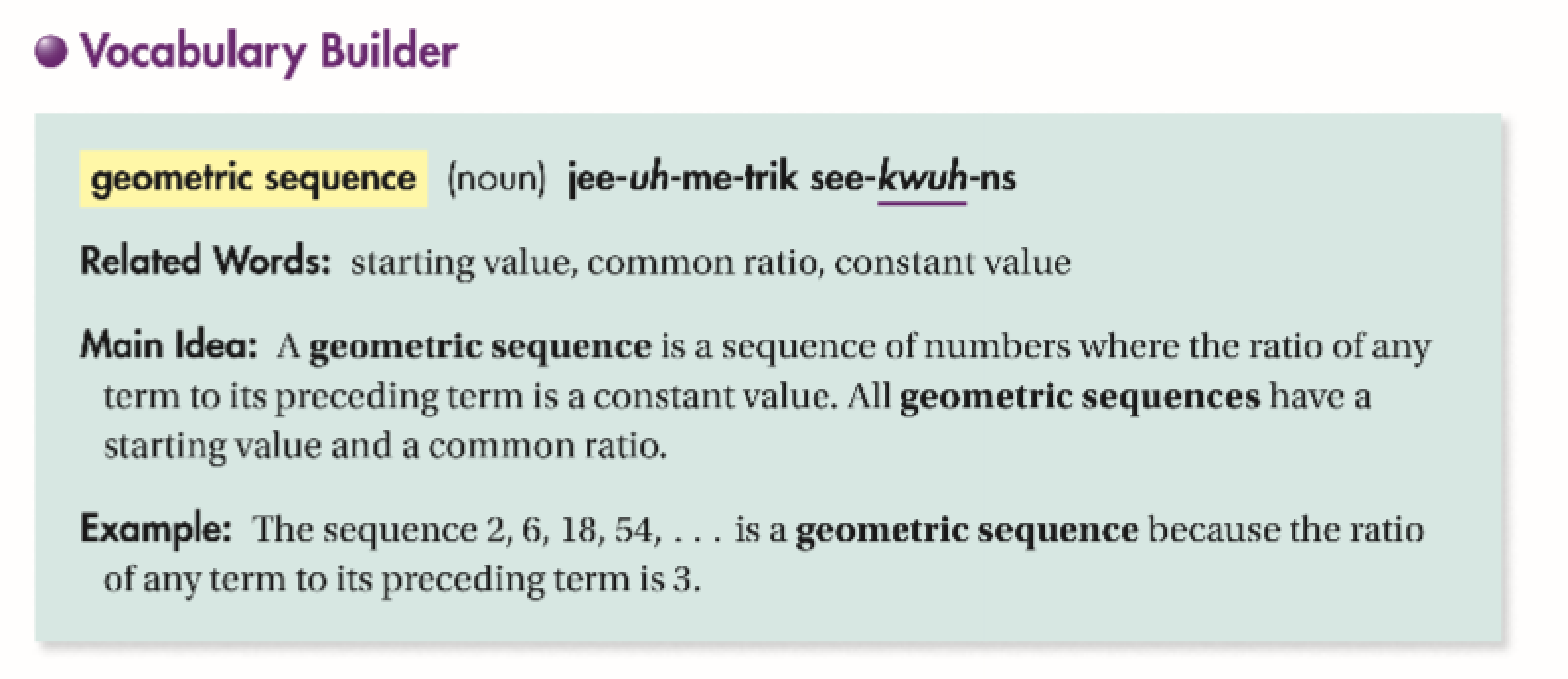29
10 pts
Use Your Vocabulary: Identify the starting value and common ratio for each geometric sequence.
• Starting value: -2
• Common ratio: -2
• Starting value: 1
• Common ratio: 2
• Starting value: 2
• Common ratio: 5
• Common ratio: -1
• 2, 4, 8, 16, 32,...
• 1, -1, 1, -1, 1,...
• 1, 5, 25, 125, 625,...
• -2, 4, -8, 16, -32,...
30
100 pts
Notes: Take a clear picture or screenshot of your Cornell notes for this lesson. Upload it to the canvas. Zoom and pan as needed.

For a refresher on the Cornell note-taking system, click here.
31
10 pts
Reflection: Math Success
Add to my formatives list

Formative uses cookies to allow us to better understand how the site is used. By continuing to use this site, you consent to the Terms of Service and Privacy Policy.